# NCERT Solutions, Matrices, Part -3, Class 12, Maths Notes - Class 12

## Class 12: NCERT Solutions, Matrices, Part -3, Class 12, Maths Notes - Class 12

The document NCERT Solutions, Matrices, Part -3, Class 12, Maths Notes - Class 12 is a part of Class 12 category.
All you need of Class 12 at this link: Class 12

Matrices and Determinants

Exercise 3.3
Question 1: Find the transpose of each of the following matrices: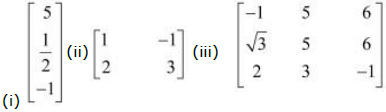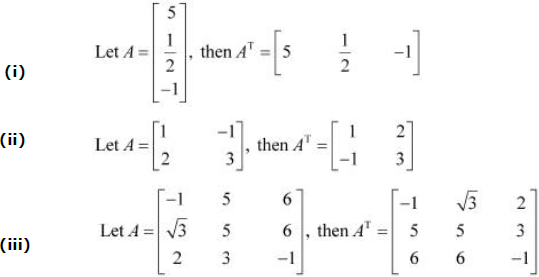Question 2: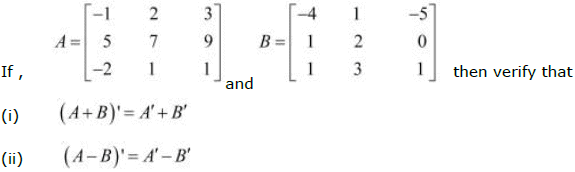We have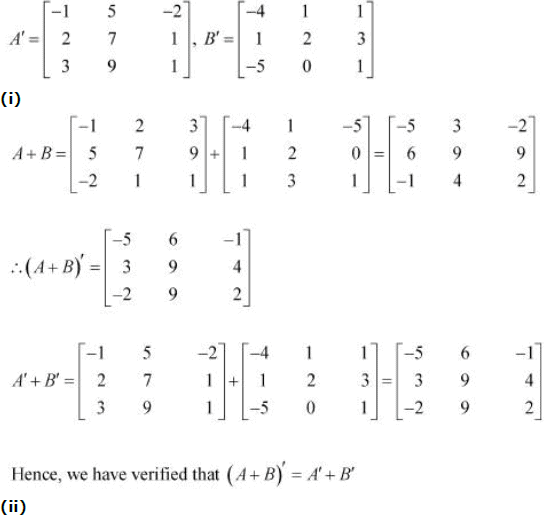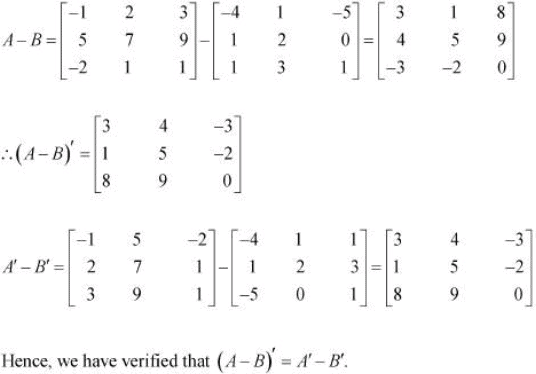Question 3: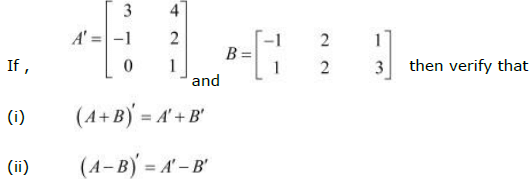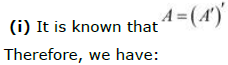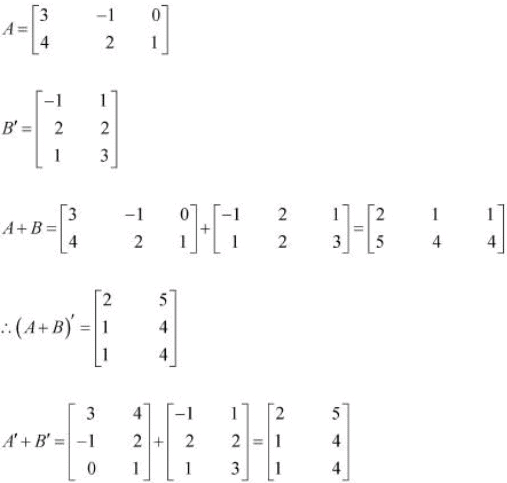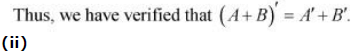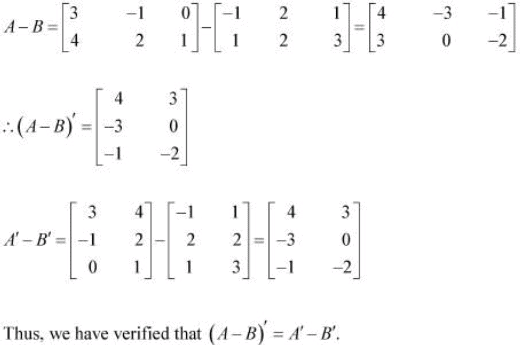Question 4: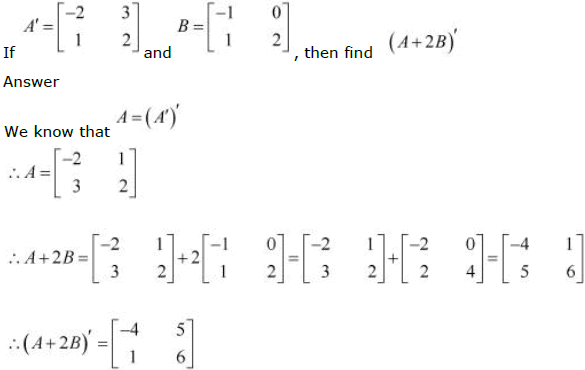Question 5: For the matrices A and B, verify that (AB)′ = B'A' where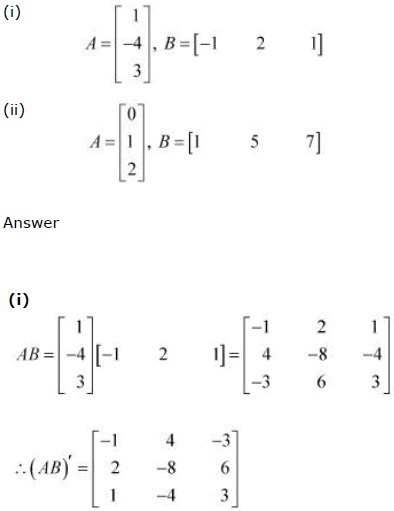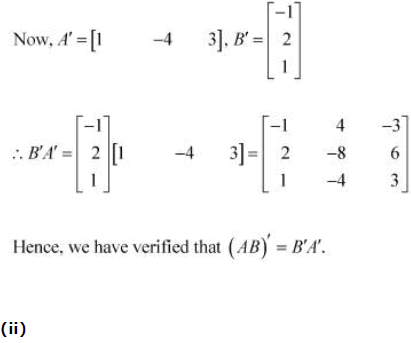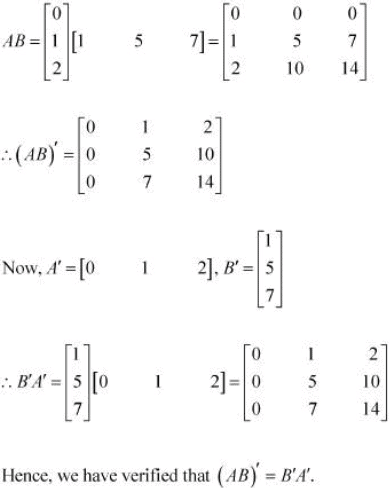Question 6: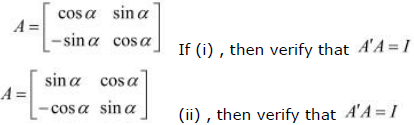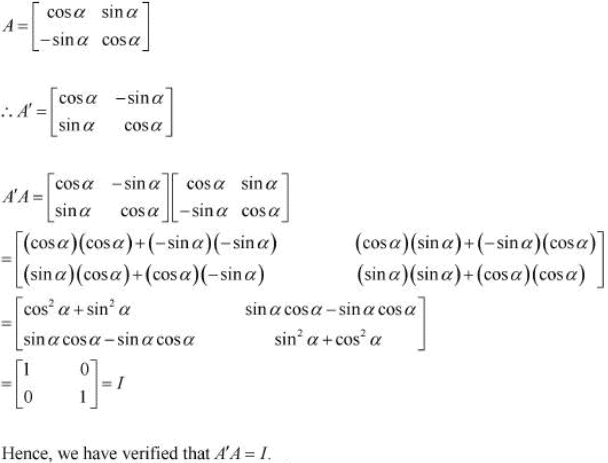(ii)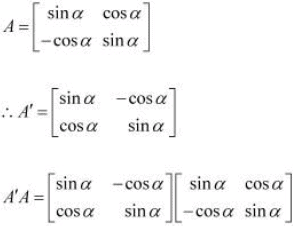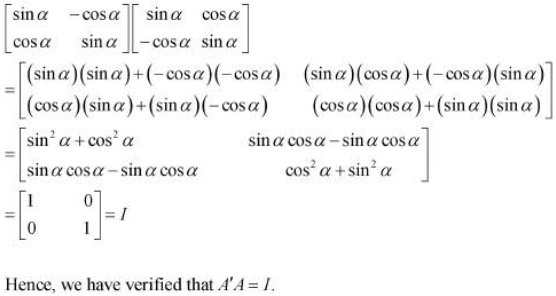Question 7: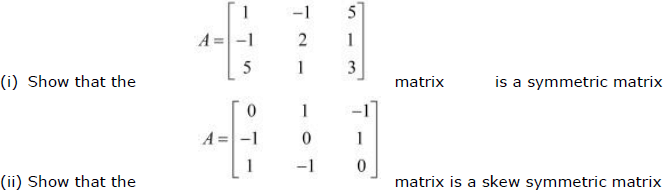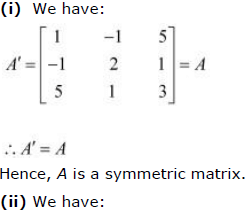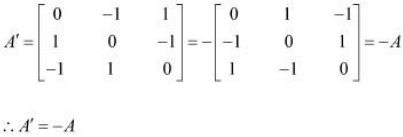Hence, A is a skew-symmetric matrix.

Question 8: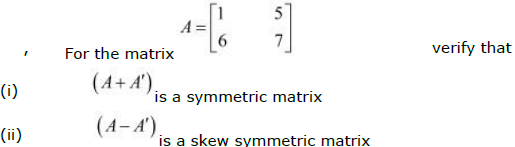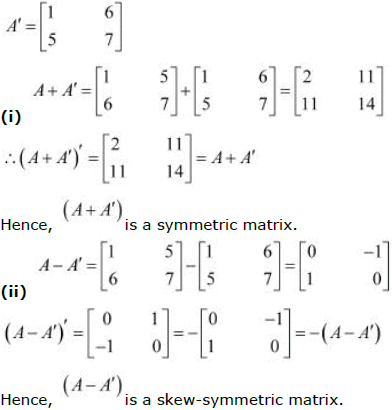Question 9: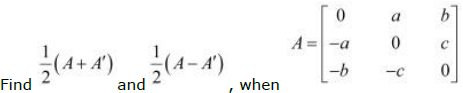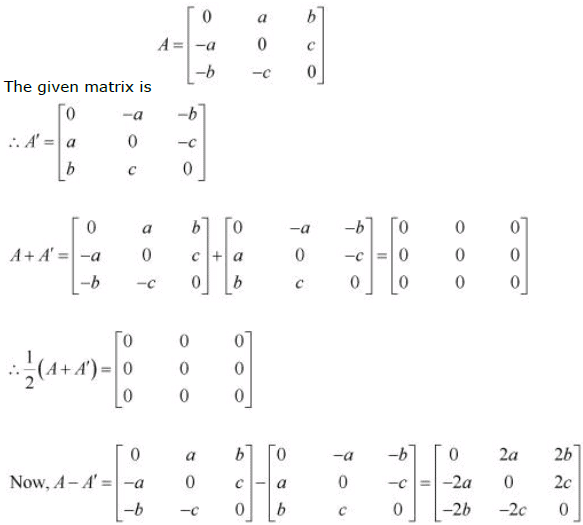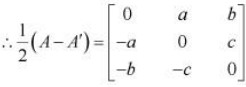Question 10: Express the following matrices as the sum of a symmetric and a skew symmetric matrix: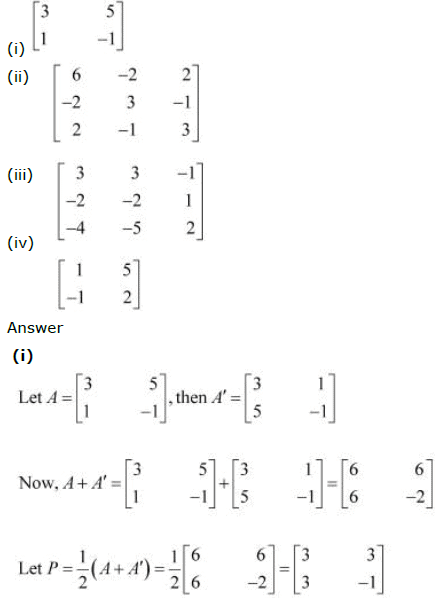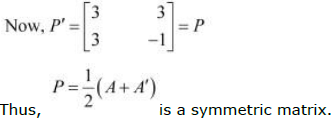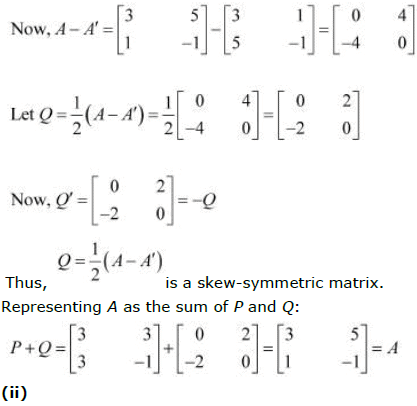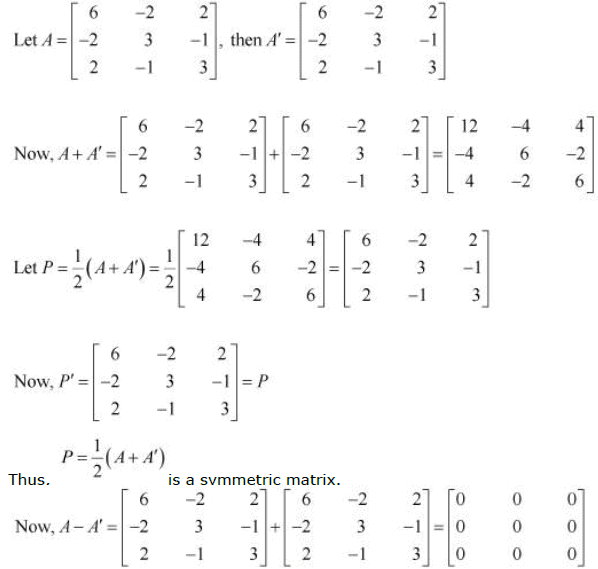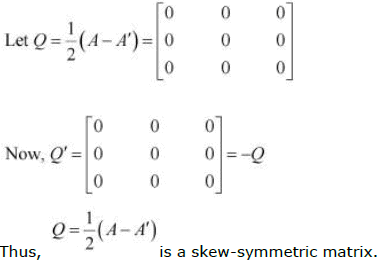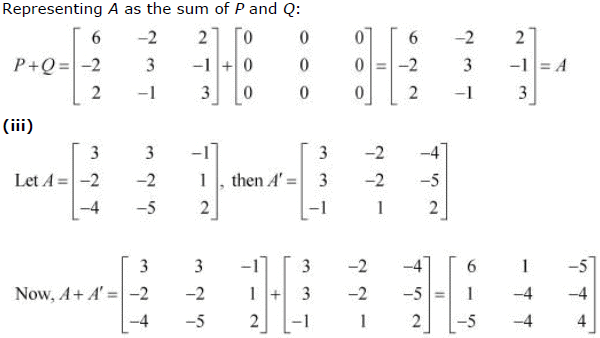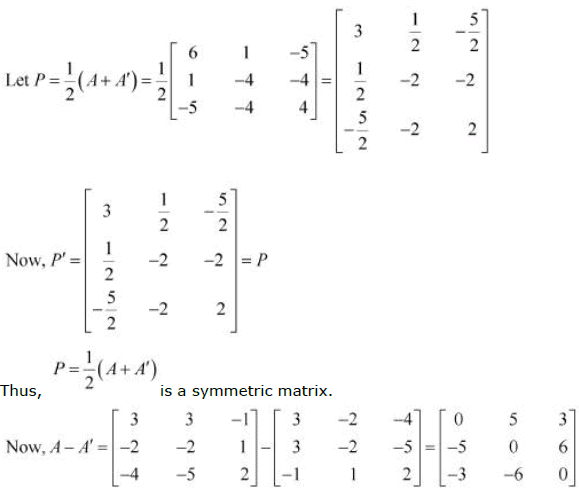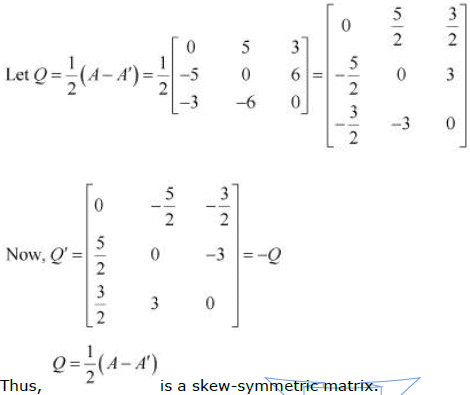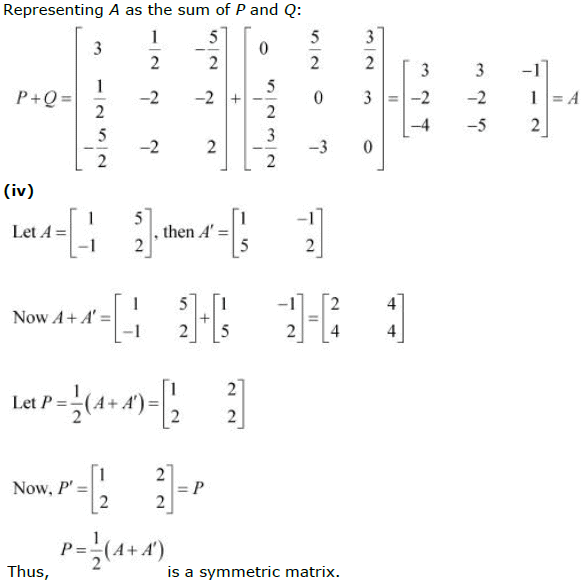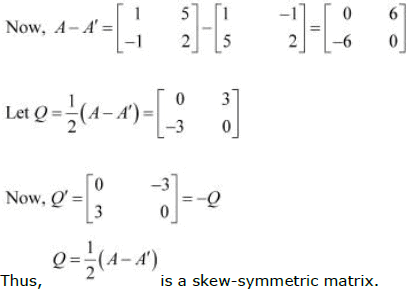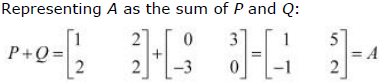Question 11: If A, B are symmetric matrices of same order, then AB − BA is a
A. Skew symmetric matrix
B. Symmetric matrix
C. Zero matrix
D. Identity matrix

A and B are symmetric matrices, therefore, we have: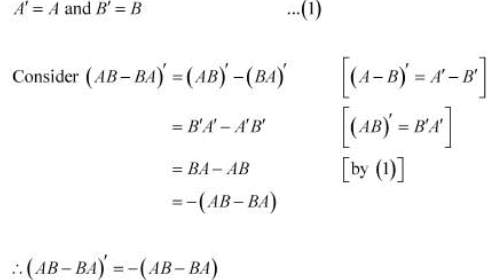Thus, (AB − BA) is a skew-symmetric matrix.

Question 12:
If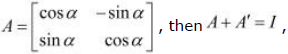if the value of α is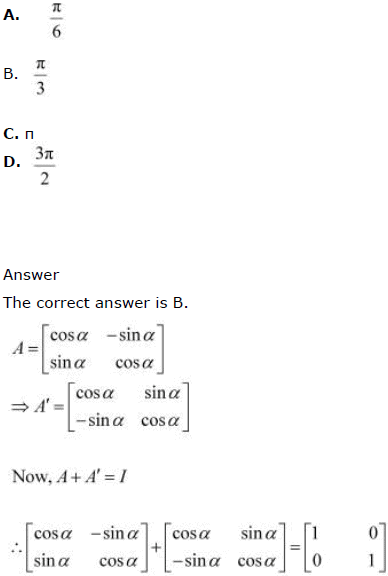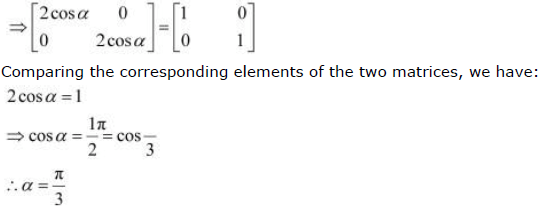The document NCERT Solutions, Matrices, Part -3, Class 12, Maths Notes - Class 12 is a part of Class 12 category.
All you need of Class 12 at this link: Class 12Use Code STAYHOME200 and get INR 200 additional OFF

Track your progress, build streaks, highlight & save important lessons and more!

,

,

,

,

,

,

,

,

,

,

,

,

,

,

,

,

,

,

,

,

,

,

,

,

,

,

,

,

,

,

,

,

,

;